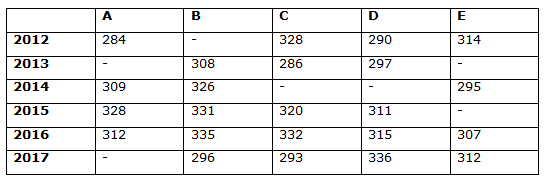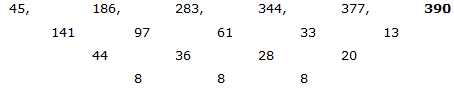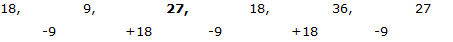# IBPS PO Mains 2018 – Quantitative Aptitude Questions Day-6

Dear Readers, Bank Exam Race for the Year 2018 is already started, To enrich your preparation here we have providing new series of Practice Questions on Quantitative Aptitude – Section. Candidates those who are preparing for IBPS PO Mains 2018 Exams can practice these questions daily and make your preparation effective.

[WpProQuiz 4016]

Directions (Q. 1 – 5): Find the wrong term in the following number series?

1) 5728, 2868, 1438, 722.25, 365.5, 186.75

a) 2868

b) 5728

c) 722.25

d) 365.5

e) 186.75

2) 250, 245, 255, 241, 260, 235

a) 241

b) 255

c) 250

d) 245

e) 260

3) 45, 186, 283, 344, 377, 389

a) 186

b) 283

c) 377

d) 389

e) 344

4) 2, 10, 47, 203, 945, 4941

a) 945

b) 203

c) 10

d) 47

e) 4941

5) 18, 9, 25, 18, 36, 27

a) 18

b) 9

c) 27

d) 36

e) 25

Directions (Q. 6 – 10) Study the following information carefully and answer the given questions:

The following table shows the number of working days of various companies in 6 different years.6) Find the difference between the number of working days of all the given companies in the year 2012 to that of 2015, if the average working days of company B in the year 2012 and 2013 is 310 days and the number of working days of company E in the year 2015 is 17 days less than the previous year?

a) 40 days

b) 20 days

c) 60 days

d) 80 days

e) None of these

7) Find the average number working days of all the given companies in the year 2014, if the ratio between the number of working days of C to that of D is 63 : 61 and the number of working days of C in the year 2014 is 5 less than that of the number of working days of C in the year 2015?

a) 325 days

b) 294 days

c) 288 days

d) 310 days

e) None of these

8) The number of working days of all the given companies in the year 2016 is approximately what percentage of the number of working days of all the given companies in the year 2017, if the ratio of number of working days of company D in the year 2013 to that of company A in the year 2017 is 9 : 10?

a) 125 %

b) 88 %

c) 102 %

d) 116 %

e) 134 %

9) Find the ratio between the number of working days of company D in the year 2014 to that of the company E in the year 2013, if the average number of working days of company D in all the given years together is 309 days and the total number of working days of company B and E in the year 2013 is 628 days?

a) 61: 64

b) 55: 57

c) 43: 49

d) 22: 25

e) None of these

10) The number of working days of company A and C in the year 2015 is approximately what percentage more/less than the number of working days of company B and E in the year 2017?

a) 20 % less

b) 7 % more

c) 20 % more

d) 7 % less

e) 30 % more

Direction (1-5) :

The correct series is,

5728, 2868, 1438, 723, 365.5, 186.75

The pattern is, ÷ 2 + 4

The wrong term is, 722.25

The correct series is,

250, 245, 255, 240, 260, 235

The pattern is, -5, + 10, -15, + 20, -25,…

The wrong term is, 241

The correct series is,The difference of difference of difference is, 8, 8, 8,…

The wrong term is, 389

The correct series is,

2, 10, 47, 205, 945, 4941

The pattern is, *1 + 23, *2 +33, *3 + 43, *4 + 53, *5 + 63,..

The wrong term is, 203

The correct series is,The alternative difference is, 9, 18,..

The wrong term is, 25

Direction (6-10) :

The average working days of company B in the year 2012 and 2013 = 310 days

The total working days of company B in the year 2012 and 2013

= > 310*2 = 620 days

The number of working days of company B in the year 2012 = 620 – 308 = 312

The number of working days of company E in the year 2015

= > The number of working days of company E in the year 2014 – 17

= > 295 – 17 = 278 days

The number of working days of all the given companies in the year 2012

= > 284 + 312 + 328 + 290 + 314 = 1528

The number of working days of all the given companies in the year 2015

= > 328 + 331 + 320 + 311 + 278 = 1568

Required difference = 1568 – 1528 = 40 days

The ratio between the number of working days of C to that of D in the year 2014 = 63 : 61

The number of working days of C in the year 2014

= > The number of working days of C in the year 2015 – 5

= > 320 – 5 = 315

63’s = 315

1’s = 5

The number of working days of D in the year 2014 = 61’s = 61*5 = 305 days

Required average = (309 + 326 + 315 + 305 + 295)/5 = 1550/5 = 310 days

The ratio of number of working days of company D in the year 2013 to that of company A in the year 2017 = 9: 10

9’s = 297

1’s = 33

The number of working days of company A in the year 2017 = 10’s = 330 days

The number of working days of all the given companies in the year 2016

= > 312 + 335 + 332 + 315 + 307 = 1601

The number of working days of all the given companies in the year 2017

=> 330 + 296 + 293 + 336 + 312 = 1567

Required % = (1601/1567)*100 = 102 %

The average number of working days of company D in all the given years together = 309 days

The total number of working days of company D in all the given years together = 309*6 = 1854

The number of working days of company D in the year 2014

= > 1854 – (290 + 297 + 311 + 315 + 336)

= > 1854 – 1549 = 305 days

The total number of working days of company B and E in the year 2013 = 628

The number of working days of company E in the year 2013

= > 628 – 308 = 320 days

Required ratio = 305: 320 = 61: 64

The number of working days of company A and C in the year 2015

= > 328 + 320 = 648 days

The number of working days of company B and E in the year 2017

= > 296 + 312 = 608 days

Required % = [(648 – 608)/608]*100 = 7 % more

Daily Practice Test Schedule | Good Luck

 Topic Daily Publishing Time Daily News Papers & Editorials 8.00 AM Current Affairs Quiz 9.00 AM Current Affairs Quiz (Hindi) 9.30 AM IBPS PO Mains – Reasoning 10.00 AM IBPS PO Mains– Quantitative Aptitude 11.00 PM Vocabulary (Based on The Hindu) 12.00 PM IBPS PO Mains – English Language 1.00 PM IBPS PO/Clerk – GK 3.00 PM Daily Current Affairs Updates 5.00 PM IBPS Clerk Prelims – Reasoning 6.00 PM IBPS Clerk Prelims – Reasoning (Hindi) 6.30 PM IBPS Clerk Prelims – Quantitative Aptitude 7.00 PM IBPS Clerk Prelims – Quantitative Aptitude (Hindi) 7.30 PM IBPS Clerk Prelims – English Language 8.00 PM